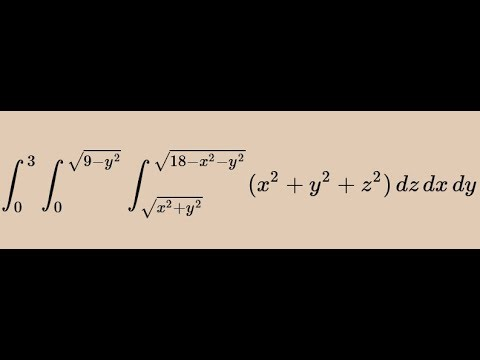# evaluate [-1,1], [0, sqrt(1-x^2)], [sqrt(x^2+y^2), sqrt(2-x^2-y^2]      sqrt(x^2+y^2+z^2)      dz dy dx ans. pi[1- sqrt(2)/2]?

I tried evaluating it through conversion to polar coordinates with the order: dx dr d(theta) ---- but I always get stuck on the "dr" part.